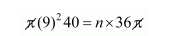# Lead spheres of diameter 6 cm are dropped into a cylindrical`
Question:

Lead spheres of diameter 6 cm are dropped into a cylindrical beaker containing some water and are fully submerged. If the diameter of the beaker is 18 cm and water rises by 40 cm. find the number of lead spheres dropped in the water.

Solution:

Radius of sphere $=\frac{6}{2}=3 \mathrm{~cm}$

Volume of lead sphere $=\frac{4}{3} \pi r^{3}$

$=\frac{4}{3} \pi(3)^{3}$

$=\frac{4}{3} \pi \times 27$

$=36 \pi \mathrm{cm}^{3}$

Let n be the no. of spheres are fully submerged.

Radius of cylinder beaker, $r_{1}=\frac{18}{2}$

$=9 \mathrm{~cm}$

and height of water raised = 40 cm

Clear,

The volume of raised water = n × volume of a sphere$n=\frac{81 \times 40}{36}$

$=90$

$n=90$

Hence, no. of lead sphere = 90